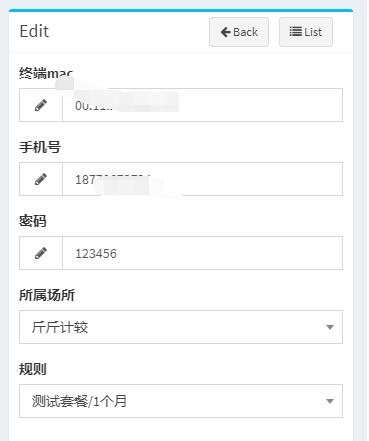更新時間：2019年10月03日 19:39:11   作者：謝高升我要評論

protected function form()
{
return Admin::form(Member::class, function (Form \$form) {

// \$form->display('id', 'ID');
\$form->text('mac', '終端mac');
\$form->text('phone', '手機號');
//判斷是不是edit
\$id = Request::route('member');
\$place_id ='';
\$rule_id = '';
if (\$id)
{
\$model = \$form->model()->find(\$id);
\$place_id = \$model->place_id;
\$rule_id = \$model->rule_id;
}
\$form->select('place_id','所屬場所')->options("/api/getPlace/\$place_id");
\$form->select('rule_id', '規則')->options("/api/getRule/\$rule_id");
});
}

//api的實現
public function getPlace(Request \$request)
{
\$name = \$request->get('p');
\$id = \$request->id;
\$where = [];
if (\$name)
{
\$where = ['name','like',"%\$name%"];
}
\$places = Place::where(\$where)->get();
\$result = [];
foreach (\$places as \$key=>\$value)
{
\$result[\$key]['id'] = \$value->Id;
\$result[\$key]['text'] = \$value->name;
if (\$value->Id == \$id)
{
\$result[\$key]['selected'] = true;
}
}
if (!\$id)
{
array_unshift(\$result,['id'=>' ','text'=>'請選擇場所','selected'=>true]);
}else{
array_unshift(\$result,['id'=>' ','text'=>'請選擇場所']);
}
return response()->json(\$result);
}